### Surface Area And Volume (cube-cuboid) Class 8th Mathematics AP Board Solution

##### Question 1.Find the volume of the cuboid whose dimensions are given below.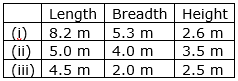Answer:i: Length = 8.2 m Breadth = 5.3m and Height = 2.6mVolume of cuboid = Length × Breadth × Height= 8.2 × 5.3 × 2.6= 112.996 m3ii: Length = 5.0 m Breadth = 4.0m and Height = 3.5mVolume of cuboid = Length × Breadth × Height= 5 × 4 × 3.5= 70 m3iii: Length = 4.5 m Breadth = 2m and Height = 2.5mVolume of cuboid = Length × Breadth × Height= 4.5 × 2 × 2.5= 22.5 m3Question 2.Find the capacity of the tanks with the following internal dimensions. Express the capacity in cubic meters and liters for each tank.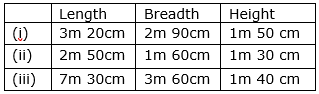Answer:i: Length = 3m 20cm = 3.2mBreadth = 2m 90cm = 2.9mHeight = 1m 50cm = 1.5mVolume of cuboid = Length × Breadth × Height= 3.2 × 2.9 × 1.5= 13.92 m3ii: Length = 2m 50cm = 2.5mBreadth = 1m 60cm = 1.6mHeight = 1m 30cm = 1.3mVolume of cuboid = Length × Breadth × Height= 2.5 × 1.6 × 1.3= 5.2 m3iii: Length = 7m 30cm = 7.3mBreadth = 3m 60cm = 3.6mHeight = 1m 40cm = 1.4mVolume of cuboid = Length × Breadth × Height= 7.3 × 3.6 × 1.4= 36.792 m3Question 3.What will happen to the volume of a cube if the length of its edge is reduced to half? Is the volume get reduced? If yes, how much?Answer:Let x be the length of the cubeVolume of cube = (side)3= x3If the length of its edge is reduced to half, then length would be =Now, Volume of cube =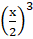=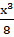Yes, the volume gets reduced bym3.Question 4.Find the volume of each of the cube whose sides are.(i) 6.4 cm (ii) 1.3 m (iii) 1.6 m.Answer:(i) Side = 6.4cmVolume of cube = (side)3= (6.4) 3= 6.4 × 6.4 × 6.4= 262.144 cm3(ii) Side = 1.3cmVolume of cube = (side)3= (1.3) 3= 1.3 × 1.3 × 1.3= 2.197 m3(iii) Side = 1.6mVolume of cube = (side)3= (1.6) 3= 1.6 × 1.6 × 1.6= 4.096 m3Question 5.How many bricks will be required to build a wall of 8 m long, 6m height and 22.5 cm thick, if each brick measures 25 cm by 11.25 cm by 6 cm?Answer:Measurements of Wall:Length = 800cm Breadth = 600cm and Height = 22.5cmVolume of wall = Length × Breadth × Height= 800 × 600 × 22.5Measurements of Brick:Length = 25cm Breadth = 11.25cm and Height = 6cmVolume of wall = Length × Breadth × Height= 25 × 11.25 × 6Number of bricks required build a wall =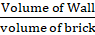=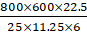= 32 × 100 × 2= 64 × 100= 6400Hence, 6400 bricks are required to build the wall.Question 6.A cuboid is 25 cm long, 15 cm broad, and 8 cm high. How much of its volume will differ from that of a cube with the edge of 16 cm?Answer:Measurement of cuboidLength = 25 cm Breadth = 15 cm and Height = 8 cmVolume of cuboid = Length × Breadth × Height= 25 × 15 × 8= 3000 cm3Measurement of cubeSide = 16cmVolume of cube = (side)3= (16) 3= 16 × 16 × 16= 4096 cm3Difference between the volumes = 4096 – 3000 cm3 = 1096 cm3Volume of cuboid differs from volume of cube by 1096 cm3.Question 7.A closed box is made up of wood which is 1cm thick. The outer dimensions of the box is 5 cm × 4 cm × 7 cm. Find the volume of the wood used.Answer:Measurement of box from outside:Length = 5 cm Breadth = 4 cm and Height = 7 cmVolume of cuboid = Length × Breadth × Height= 5 cm × 4 cm × 7 cm= 140 cm3Measurement of box from the insideLength = 4 cm Breadth = 3 cm and Height = 6 cmVolume of cuboid = Length × Breadth × Height= 4 cm × 3 cm × 6 cm= 72 cm3Volume of wood used = volume of the box from outside – volume of the box from inside= 140 – 72= 68 cm3Question 8.How many cubes of edge 4cm, each can be cut out from cuboid whose length, breadth and height are 20 cm, 18 cm and 16 cm respectively.Answer:Measurement of cuboidLength = 20 cm Breadth = 18 cm and Height = 16 cmVolume of cuboid = Length × Breadth × Height= 20 × 18 × 16= 5760 cm3Measurement of cubeSide = 4 cmVolume of cube = (side)3= (4) 3= 4 × 4 × 4= 64 cm3Number of cube which can be cut from the cuboid =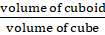=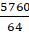=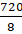= 9090 cubes can be cut out from the cuboid.Question 9.How many cuboids of size 4 cm × 3 cm × 2 cm can be made from a cuboid of size 12 cm × 9 cm × 6 cm?Answer:Measurement of bigger cuboidLength = 12 cm Breadth = 9 cm and Height = 6 cmVolume of cuboid = Length × Breadth × Height= 12cm × 9cm × 6cmMeasurement of smaller cuboidLength = 4 cm Breadth = 3 cm and Height = 2 cmVolume of cuboid = Length × Breadth × Height= 4cm × 3cm × 2cmNumber of smaller cuboids which can be made from bigger cuboid=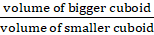=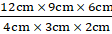= 3 × 3 × 3= 27 cuboids27 cuboids of smaller cuboids can be made from bigger cuboid.Question 10.A vessel in the shape of a cuboid is 30 cm long and 25 cm wide. What should be its height to hold 4.5 liters of water?Answer:We know that,1 liter is 1000cm3,∴ 4.5 liters = 4.5 × 1000 = 4500 cm3Measurement of vesselLength = 30 cm,Breadth = 25cm,And Height =?Let the height be h cm.We are given the capacity which this vessel can hold i.e. the volume of the vessel.Volume of vessel = Length × Breadth × Height4500 = 300 × 25 × h cm4500 = 750 × hh =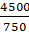h =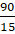h = 6 cmHence, height of the vessel is 6cm which can hold 4.5 liters.

PDF FILE TO YOUR EMAIL IMMEDIATELY PURCHASE NOTES & PAPER SOLUTION. @ Rs. 50/- each (GST extra)

HINDI ENTIRE PAPER SOLUTION

MARATHI PAPER SOLUTION

SSC MATHS I PAPER SOLUTION

SSC MATHS II PAPER SOLUTION

SSC SCIENCE I PAPER SOLUTION

SSC SCIENCE II PAPER SOLUTION

SSC ENGLISH PAPER SOLUTION

SSC & HSC ENGLISH WRITING SKILL

HSC ACCOUNTS NOTES

HSC OCM NOTES

HSC ECONOMICS NOTES

HSC SECRETARIAL PRACTICE NOTES

# 2019 Board Paper Solution

HSC ENGLISH SET A 2019 21st February, 2019

HSC ENGLISH SET B 2019 21st February, 2019

HSC ENGLISH SET C 2019 21st February, 2019

HSC ENGLISH SET D 2019 21st February, 2019

SECRETARIAL PRACTICE (S.P) 2019 25th February, 2019

HSC XII PHYSICS 2019 25th February, 2019

CHEMISTRY XII HSC SOLUTION 27th, February, 2019

OCM PAPER SOLUTION 2019 27th, February, 2019

HSC MATHS PAPER SOLUTION COMMERCE, 2nd March, 2019

HSC MATHS PAPER SOLUTION SCIENCE 2nd, March, 2019

SSC ENGLISH STD 10 5TH MARCH, 2019.

HSC XII ACCOUNTS 2019 6th March, 2019

HSC XII BIOLOGY 2019 6TH March, 2019

HSC XII ECONOMICS 9Th March 2019

SSC Maths I March 2019 Solution 10th Standard11th, March, 2019

SSC MATHS II MARCH 2019 SOLUTION 10TH STD.13th March, 2019

SSC SCIENCE I MARCH 2019 SOLUTION 10TH STD. 15th March, 2019.

SSC SCIENCE II MARCH 2019 SOLUTION 10TH STD. 18th March, 2019.

SSC SOCIAL SCIENCE I MARCH 2019 SOLUTION20th March, 2019

SSC SOCIAL SCIENCE II MARCH 2019 SOLUTION, 22nd March, 2019

XII CBSE - BOARD - MARCH - 2019 ENGLISH - QP + SOLUTIONS, 2nd March, 2019

# HSCMaharashtraBoardPapers2020

(Std 12th English Medium)

HSC ECONOMICS MARCH 2020

HSC OCM MARCH 2020

HSC ACCOUNTS MARCH 2020

HSC S.P. MARCH 2020

HSC ENGLISH MARCH 2020

HSC HINDI MARCH 2020

HSC MARATHI MARCH 2020

HSC MATHS MARCH 2020

# SSCMaharashtraBoardPapers2020

(Std 10th English Medium)

English MARCH 2020

HindI MARCH 2020

Hindi (Composite) MARCH 2020

Marathi MARCH 2020

Mathematics (Paper 1) MARCH 2020

Mathematics (Paper 2) MARCH 2020

Sanskrit MARCH 2020

Sanskrit (Composite) MARCH 2020

Science (Paper 1) MARCH 2020

Science (Paper 2)

Geography Model Set 1 2020-2021

MUST REMEMBER THINGS on the day of Exam

Are you prepared? for English Grammar in Board Exam.

Paper Presentation In Board Exam

How to Score Good Marks in SSC Board Exams

Tips To Score More Than 90% Marks In 12th Board Exam

How to write English exams?

How to prepare for board exam when less time is left

How to memorise what you learn for board exam

No. 1 Simple Hack, you can try out, in preparing for Board Exam

How to Study for CBSE Class 10 Board Exams Subject Wise Tips?

JEE Main 2020 Registration Process – Exam Pattern & Important Dates

NEET UG 2020 Registration Process Exam Pattern & Important Dates

How can One Prepare for two Competitive Exams at the same time?

8 Proven Tips to Handle Anxiety before Exams!